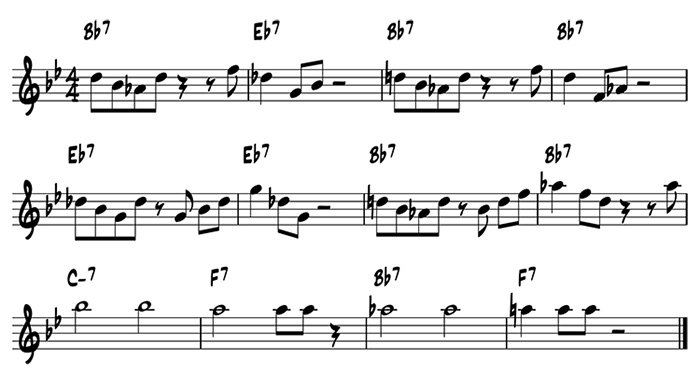Lesson 11: Chord Tone Solos on the Blues
Focus on Harmony

## Blues solos using chord tones

Lesson 11 applies the chord tone approach of Lesson 10 to the B flat blues.  In this assignment, you will use repetition, variation, and contrast with a choice of all chord tones on the blues chord progression.

Lesson 11 Assignment

### Part 1: Compose

Compose a solo that follows these guidelines, with the option of using chords tones in each measure:

In measures 1 and 2, compose a two-measure idea using any of the chord tones for each measure.
In measures 3 and 4, compose an idea that repeats the rhythm and contour of the idea in measures 1 and 2 (the pitches might not be the same because the chords are different).
In measures 5 and 6, compose a variation on the idea of measures 3 and 4.
In measures 7 and 8, compose a variation on the idea of measures 5 and 6.
In measures 9 and 10, compose a contrast to the idea of measures 7 and 8.
In measures 11 and 12, compose a variation on the idea of measures 9 and 10.Part 2: Memorize

Memorize the solo that you have written.

Part 3: Improvise

Improvise a solo following the same guidelines as your written solo.

Lesson 12: Chord Tone Solos with an Emphasis on Guide Tones